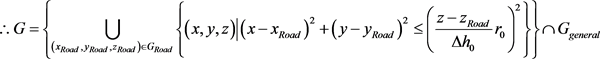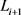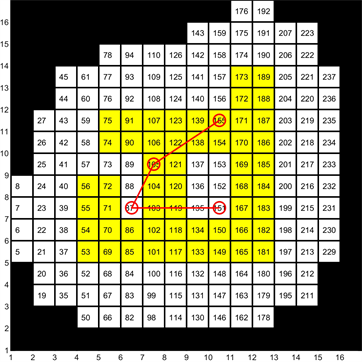﻿ 一种无人机覆盖路径规划问题的算法

# 一种无人机覆盖路径规划问题的算法An Algorithm for Coverage Path Planning with Unmanned Aerial Vehicles

Abstract: The application of Unmanned Aerial Vehicles in road monitoring of disaster emergency rescue system which enables rescue organizations to obtain road network conditions in time provides more accurate ground information and improves rescue efficiency. Monitoring road by unmanned aerial vehicles needs to path planning according to the characteristics of remote sensing imaging and time is an important index of disaster emergency rescue system design. In order to speed up the solution of track planning, a set coverage model is established considering the characteristics of road monitoring in disaster emergency rescue system. A three-stage algorithm is proposed to decouple the problem into two subproblems. Finally, a concrete example is given to verify the fea-sibility of the algorithm.

1. 引言

2. 问题描述及模型分析

${G}_{{F}_{i},Monitor}=\underset{j=1}{\overset{\infty }{\cup }}\left\{\left(x,y,z\right)|{\left(x-{x}_{i,0}\right)}^{2}+{\left(y-{y}_{i,0}\right)}^{2}\le {\left(\frac{{z}_{i,0}-{z}_{road}}{\Delta {h}_{0}}{r}_{0}\right)}^{2},\left({x}_{i,j},{y}_{i,j},{z}_{i,j}\right)\in {F}_{i}\right\}$ .$\left({x}_{Road},{y}_{Road},{z}_{Road}\right)\in G$

$\exists \left({x}_{i,j},{y}_{i,j},{z}_{i,j}\right)\in {F}_{i},\text{\hspace{0.17em}}s.t.\text{\hspace{0.17em}}{\left({x}_{Road}-{x}_{i,j}\right)}^{2}+{\left({y}_{Road}-{y}_{i,j}\right)}^{2}\le {\left(\frac{{z}_{i,j}-{z}_{Road}}{\Delta {h}_{0}}{r}_{0}\right)}^{2}$${G}^{C}={G}_{general}-G=\underset{\left({x}_{Road},{y}_{Road},{z}_{Road}\right)\notin {G}_{Road}}{\cup }\left\{\left(x,y,z\right)|{\left(x-{x}_{Road}\right)}^{2}+{\left(y-{y}_{Road}\right)}^{2}\le {\left(\frac{z-{z}_{Road}}{\Delta {h}_{0}}{r}_{0}\right)}^{2}\right\}\ne \varnothing$

3. 近似算法研究

STEP1：对区域Ggeneral使用栅格法进行空间的划分，以道路所在单元中心为圆心画圆确定G，将 ${G}_{1}=G$ 所在的单元视为LSCP问题中的候选拍摄点，建立整数规划模型。使用分支定界法求解，并确定 circle_min。

STEP2：检查STEP1中求出的 $circle_\mathrm{min}$ 个拍摄点，若存在两点之间的距离小于 $\Delta s={v}_{UAV}t$，( ${v}_{UAV}$ 为无人机的速度， $\Delta s$ 为无人机拍摄两张照片所需的最小飞行间距，在一般情况下，如果 ${L}_{i},{L}_{i+1}$ 两点间的距离小于 $\Delta s$，可以降低无人机的速度，使得无人机从 ${L}_{i}$ 飞到时，相机能够拍照，但是降低速度无疑会使完成任务的时间变长，而灾害救援系统有时间限制)，则从 ${G}_{1}$ 删去处于更“稠密”区域的那点。返回到STEP1重新计算。如果所有点的距离均不小于 $\Delta s$，则计算当前方案的总权重，执行STEP3。

STEP3：使用启发式算法求解出满足预定精度的方案或使用遍历算法得到最优方案。

STEP4：求解出访问STEP3中求出的最优方案中 $circle_\mathrm{min}$ 个单元的先后顺序。

4. 算例研究Figure 2. The solution result of path

5. 结束语

NOTES

*第一作者。

 夏威. 交通道路灾害监测与应急响应系统的研究[J]. 通讯世界, 2015(17): 245-247.

 Luo, C., Miao, W., Ullah, H., et al. (2019) Unmanned Aerial Vehicles for Disaster Management. In: Durrani, T.S., Wang, W. and Forbes, S.M., Eds., Geological Disaster Monitoring Based on Sensor Networks, Springer, Berlin, 83-107.
https://doi.org/10.1007/978-981-13-0992-2_7

 金伟, 葛宏立, 杜华强, 等. 无人机遥感发展与应用概况[J]. 遥感信息, 2009(1): 88-92.

 巴海涛. 无人机航迹规划研究[D]: [硕士学位论文]. 西安: 西北工业大学, 2006.

 郑昌文, 严平, 丁明跃, 等. 飞行器航迹规划研究现状与趋势[J]. 宇航学报, 2007(6): 1441-1446.

 胡中华. 基于智能优化算法的无人机航迹规划若干关键技术研究[D]: [博士学位论文]. 南京: 南京航空航天大学, 2011.

 Jang, D.S., Chae, H.J. and Choi, H.L. (2017) Optimal Control-Based UAV Path Planning with Dynamical-ly-Constrained TSP with Neighborhoods. 17th International Conference on Control, Automation and Systems, Ramada Plaza, 18-21 October 2017, 373-378.
https://doi.org/10.23919/ICCAS.2017.8204468

 Jeong, S., Simeone, O. and Kang, J. (2017) Mobile Edge Computing via a UAV-Mounted Cloudlet: Optimization of Bit Allocation and Path Planning. IEEE Transactions on Vehicular Technology, 67, 2049-2063.
https://doi.org/10.1109/TVT.2017.2706308

 San Juan, V., Santos, M. and Andújar, J.M. (2018) Intelligent UAV Map Generation and Discrete Path Planning for Search and Rescue Operations. Complexity, 2018, Article ID: 6879419.
https://doi.org/10.1155/2018/6879419

 Torres, M., Pelta, D.A., Verdegay, J.L., et al. (2016) Cov-erage Path Planning with Unmanned Aerial Vehicles for 3D Terrain Reconstruction. Expert Systems with Applications, 55, 441-451.
https://doi.org/10.1016/j.eswa.2016.02.007

 Gomez, J.I.V., Melchor, M.M. and Lozada, J.C.H. (2017) Optimal Coverage Path Planning Based on the Rotating Calipers Algorithm. International Conference on Mechatronics, Electronics and Automotive Engineering, Cuernavaca, 21-24 November 2017, 140-144.
https://doi.org/10.1109/ICMEAE.2017.11

 Cabreira, T., Brisolara, L. and Ferreira, P.R. (2019) Survey on Coverage Path Planning with Unmanned Aerial Vehicles. Drones, 3, 4.
https://doi.org/10.3390/drones3010004

Top